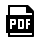Which Machine Learning Algorithm Is Right for You?

You have data and an application, but which algorithm should you try first? There are tradeoffs no matter what you choose; here are some basic principles to get you started.Small

• Decision trees
• Linear models (including logistic regression and linear discriminant)

Small

• Decision Trees
• Linear Models (including Logisting Regression Linear Discriminant)

Medium

• (Nonlinear) SVM
• Naïve Bayes
• Nearest neighbor
• Neural network (shallow)

Medium

• (Nonlinear) SVM
• Naive Bayes
• Nearest Neighbor
• Neural Network (Shallow)

• Deep nets
• Ensembles

• Deep Nets
• Ensembles